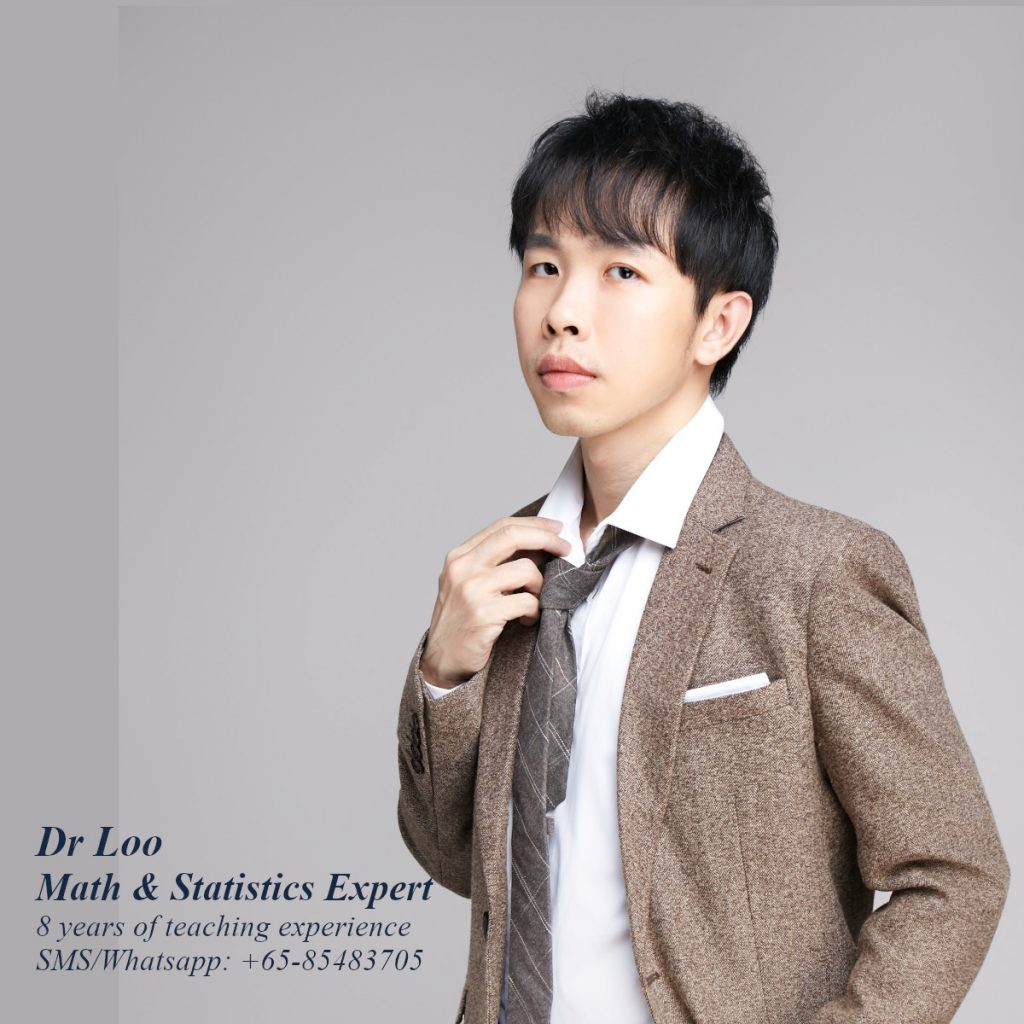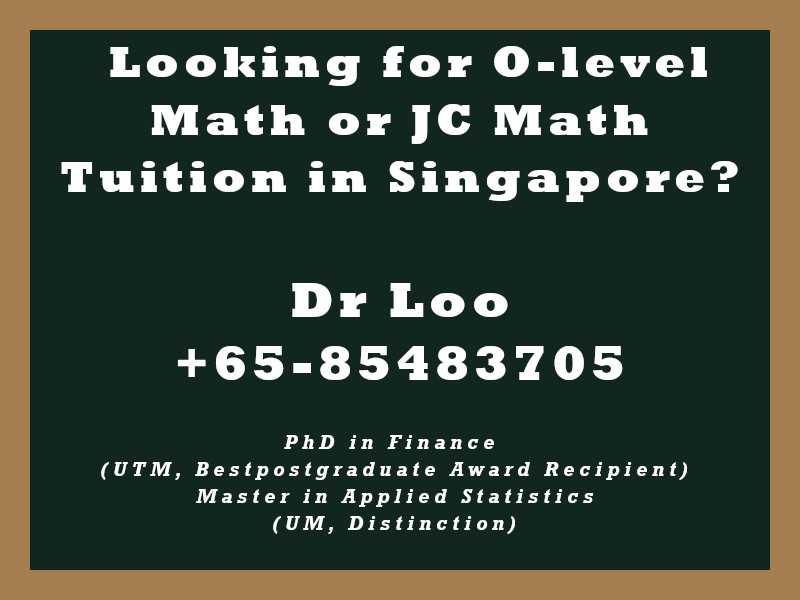+65-85483705
Select Page

# Adding & Subtracting Algebraic Fractions

Algebraic fraction represents a quotient of two algebraic expressions. Students will normally learn the use of algebraic fraction in O-level mathematics class. The use of algebraic fraction serve as a fundamental knowledge when learning A-level mathematics. When dealing with the operation of algebraic fractions, it can either involve the operation of the algebraic fractions with same denominators or algebraic fraction with different denominators.

## Things to pay attention to when dealing with algebraic fraction

1. Whether addition or subtraction, before performing the operation, the denominator of all fractions need to be expressed as common denominator.
2. For example, (x/3) + (1/4) needs to be expressed as (4x/12) + (3/12) in order to perform the operation.
3. This can be done by finding their lowest common multiple (LCM).
4. Simplify the numerator and finally simplify the algebraic fraction.

## Algebraic Fraction Practice

Question

For the following algebraic expression, simplify it.

1. (x/5) – x
2. (x/3) + (x/6)
3. (1/a) + (1/b)

1. -4x/5
2. x/2
3. (a+b)/ab

### Having difficulty in understanding the addition and substraction of algebra?

The operation of algebra is one of the important fundamental topics to be learnt in most of the secondary school math syllabus, you are encourage to look for a secondary math teacher or a O-level math tuition teacher to clarify about this topic if you have problems in understanding it.

## About the Math Tutor - Dr Loo

I am a PhD holder with 9 years of teaching experience at secondary school and university. My expertizes are mathematics, statistics, econometrics, finance and machine learning.

Currently I do provide consultation and private tuition in Singapore. For those who are looking for math tutor in Singapore or statistics tutor in Singapore, please feel free to contact me at +65-85483705 (SMS/Whatsapp/Telegram).

Some of the math tuition in Singapore I provide includes O-level math tuition and also JC Math Tuition (H1 Math Tuition & H2 Math Tuition). On the other hand, my statistics tuition in Singapore mostly focus on pre-university level until postgraduate level. For those who prefer online tutoring service, the online O-level math tuition and online JC math tuition are also available.## For those who are looking for math tution in Singapore

Need help with this topic? I do provide mathematics home tuition in Singapore for O-level math and also JC H2 math. In addition, online math tutoring is available as well. Feel free to contact me if you would like to know further.